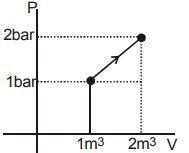Courses
Courses for Kids
Free study material
Offline Centres
MoreLast updated date: 07th Dec 2023
Total views: 280.2k
Views today: 2.80k

# What is $\Delta U$ for the process described by figure? Heat supplied during the process $q = 100kJ$.A.+50 kJB.-50 kJC.+150 kJD.-150 kJVerified
280.2k+ views
Hint: The first law of thermodynamics extends the conservation of energy theory to systems in which energy is transferred into and out of the system by heat transfer and work. The change in internal energy of a system equals the net heat flow into the system minus the net work performed by the system, according to the first law of thermodynamics. The first law of thermodynamics is written as $\Delta U{\text{ }} = {\text{ }}Q{\text{ }} - \;W$ in equation form.

The shift in the system's internal energy U is denoted by $\Delta U$. The number of all heat flow into and out of the system is Q, which is the net heat transmitted into the system. W denotes the system's network, or the amount of all work performed on or by the system. We use the following sign conventions: if Q is positive, the system is transferring net heat; if W is positive, the system is transferring network. As a result, positive Q contributes energy to the system when positive W depletes it. It's also worth noting that if the device absorbs more heat than it produces, the difference is retained as internal energy.
As the Carnot cycle is depicted as a graph of gas pressure versus volume (a p-V diagram), we can see how much work is completed in a full cycle.
Remember that $w{\text{ }} = {\text{ }}p{\text{ }}\Delta V$equals the work performed when a gas changes volume at constant pressure. A region on a p-V diagram represents the product of pressure and volume. The work performed during the process is represented by the region under the curve on a p-V diagram.
q=100 kJ (given)
$\Delta V$=?
$\Delta U = q + w$ (From first law of thermodynamics)
w= Area under P−V curve
$w = \dfrac{1}{2}(1 + 2) \times 1$
$w = \dfrac{3}{2}bar - {m^3}$
$w = \dfrac{3}{2} \times 100k$
w=150 kJ
$\ \Delta U = - 100{\text{ }}kJ + 150{\text{ }}kJ \\ \Delta U{\text{ }} = {\text{ }} + 50{\text{ }}kJ \\$
Hence, option A is correct.

Note:
Any thermodynamic system exists in a specific state. A thermodynamic loop occurs when a system is taken through a sequence of various states before being returned to its original state. The machine can perform work on its surroundings while going through this loop, for example, by moving a piston and acting as a heat engine. A Carnot heat engine is a machine that goes through the Carnot loop, but such a "perfect" engine is just a theoretical concept that cannot be designed in reality. A microscopic Carnot heat engine, on the other hand, has been developed and tested.